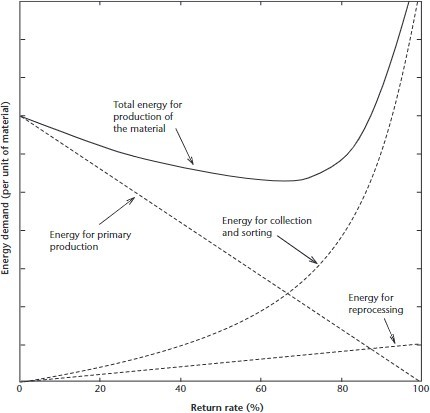Home / Expert Answers / Earth Sciences / the-figure-below-is-a-qualitative-representation-of-the-total-energy-required-for-recycling-pa770

# (Solved): The figure below is a qualitative representation of the total energy required for recycling. ...

The figure below is a qualitative representation of the total energy required for recycling.???????

What does the return rate mean? Describe it in words, and also with an equation The energy demand per unit or material is heavily influenced by the energy for collection and sorting.

Why does this curve increase non-linearly with return rate?

In the figure above, what is the optimal return rate from the perspective of minimising the energy demand of a material?

We have an Answer from Expert